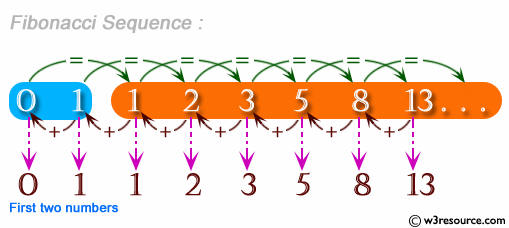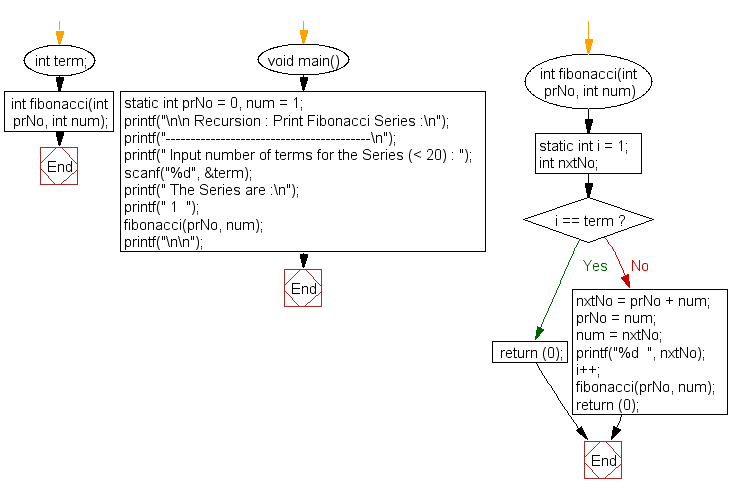﻿ C Program: Print Fibonacci Series - w3resource

# C Exercises: Print Fibonacci Series

## C Recursion : Exercise-3 with Solution

Write a program in C to print the Fibonacci Series using recursion.

Pictorial Presentation:Sample Solution:

C Code:

``````#include<stdio.h>

int term;
int fibonacci(int prNo, int num);

void main()
{
static int prNo = 0, num = 1;
printf("\n\n Recursion : Print Fibonacci Series :\n");
printf("-----------------------------------------\n");

printf(" Input number of terms for the Series (< 20) : ");
scanf("%d", &term);
printf(" The Series are :\n");
printf(" 1  ");
fibonacci(prNo, num);
printf("\n\n");
}

int fibonacci(int prNo, int num)
{
static int i = 1;
int nxtNo;

if (i == term)
return (0);
else
{
nxtNo = prNo + num;
prNo = num;
num = nxtNo;
printf("%d  ", nxtNo);

i++;
fibonacci(prNo, num); //recursion, calling the function fibonacci itself
}
return (0);
}
```
```

Sample Output:

``` Recursion : Print Fibonacci Series :
-----------------------------------------
Input number of terms for the Series (< 20) : 10
The Series are :
1  1  2  3  5  8  13  21  34  55
```

Explanation:

```int fibonacci(int prNo, int num)
{
static int i = 1;
int nxtNo;

if (i == term)
return (0);
else
{
nxtNo = prNo + num;
prNo = num;
num = nxtNo;
printf("%d  ", nxtNo);

i++;
fibonacci(prNo, num); //recursion, calling the function fibonacci itself
}
return (0);
}
```

The above Fibonacci() function calculates and prints the Fibonacci series up to a certain term, using recursion. It takes two integer parameters ‘prNo’ and ‘num’, representing the previous and current numbers in the series, respectively. The function starts by checking if the current term i has reached the desired term (term is not defined in the code snippet provided), and if so, it returns 0. If not, it calculates the next number in the series by adding the previous and current numbers, and then prints this number. It then updates prNo and num to reflect the next two numbers in the series, increments i, and calls itself recursively with the updated parameters.

Time complexity and space complexity:

The time complexity of the fibonacci function is O(n), where n is the term up to which the series is being calculated. This is because each term in the series requires a constant amount of time to be computed, and the function computes n terms.

The space complexity is O(1), since the function uses only a constant amount of additional space for the variables prNo, num, and i.

Flowchart:C Programming Code Editor:

Have another way to solve this solution? Contribute your code (and comments) through Disqus.

What is the difficulty level of this exercise?

Test your Programming skills with w3resource's quiz.

﻿

## C Programming: Tips of the Day

__FILE__ macro shows full path

```#include <string.h>

#define __FILENAME__ (strrchr(__FILE__, '/') ? strrchr(__FILE__, '/') + 1 : __FILE__)
For Windows use '\\' instead of '/'.
```

Ref : https://bit.ly/3iEWRoT

We are closing our Disqus commenting system for some maintenanace issues. You may write to us at reach[at]yahoo[dot]com or visit us at Facebook